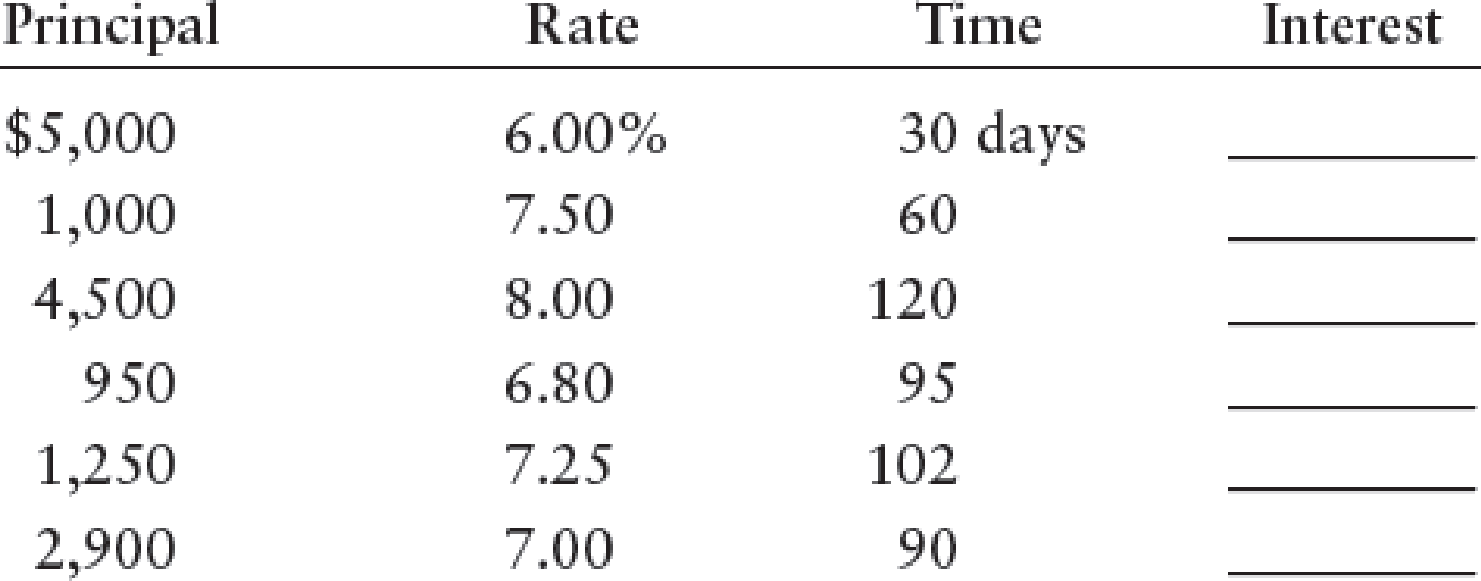Chapter 17, Problem 2SEA

Chapter
Section
Textbook Problem

CALCULATING INTEREST Using 360 days as the denominator, calculate interest for the following notes using the formula I = P × R × T.To determine

Calculate interest for the following notes.

Explanation

Accrued interest:

Accrued interest is the outstanding interest expense in the accounting period, as on the reporting date of financial statements.

Calculate interest for the following notes as follows:

 Principal (A) Rate (B) Time (C ) Interest ((A×B×C)÷360) \$5,000 6% 30 \$25 \$1,000 7...

Still sussing out bartleby?

Check out a sample textbook solution.

See a sample solution

The Solution to Your Study Problems

Bartleby provides explanations to thousands of textbook problems written by our experts, many with advanced degrees!

Get Started

Find more solutions based on key concepts